What is Gradient Descent in machine learning?

# What is Gradient Descent in machine learning?

The process of repeatedly nudging an input of a function by some multiple of the negative gradient is called gradient descent. It’s a way to converge towards some local minimum of a cost function basically valley in a graph.  According to Wikipedia, Gradient descent is a first-order iterative optimization algorithm for finding the minimum of a function. To find a local minimum or minimum cost of a function using gradient descent, one takes steps proportional to the negative of the gradient of the function at the current point.

Gradient descent is a first-order iterative optimization algorithm for finding the minimum of a function.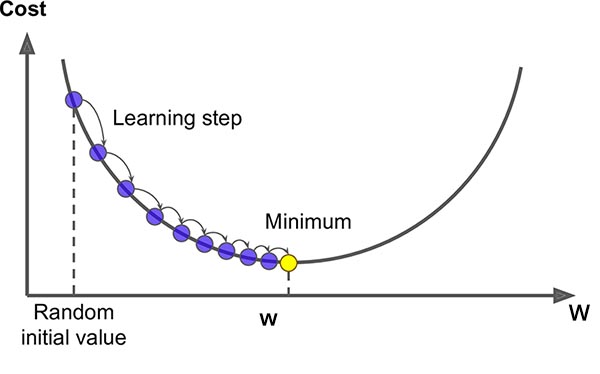Gradient Descent is an algorithm for miniming some arbitary function or cost function.

Gradient Descent is a simple optimization technique that could be used in many machine learning problems. It involves reducing the cost function. The cost function could be anything like least square methods, cross entropy. The cost function is the relation between calculated output and actual output. Here’s the image from an article of balamulari in the medium. In this experiment, balamulari is trying to find the minimum cost of function x^2.  Here, he started from (1,1) to well known minimum cost  (0,0) in the figure. He has tried various learning rate i.e. alpha = 0, 0.11111, 0.22222, 0.33333, 0.44444, 0.55556, 0.66667, 0.77778, 0.88889, 1.  At alpha = 0 there is no weight update and at alpha = 1 gets struck (it never converges as it hit the other end (-1,1)) . With alpha with 0.11111 converges very slowly and alpha with 0.66667 the fastest. And, alpha with 0.88889 oscillates several times before reaching the optimal point (0,0). If you want to learn more please visit the above mention link.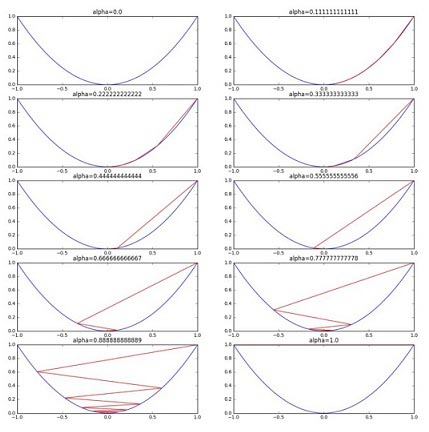Fig: learning rate in cost function = x^2

Learning rate has greater importance in gradient descent. Choosing the very small learning rate leads to many iterations until convergence and the possibility of trapping in local minima. And choosing too large learning rate could result to overshoot the optimum value. Below image gives you some insight into what I mean to say.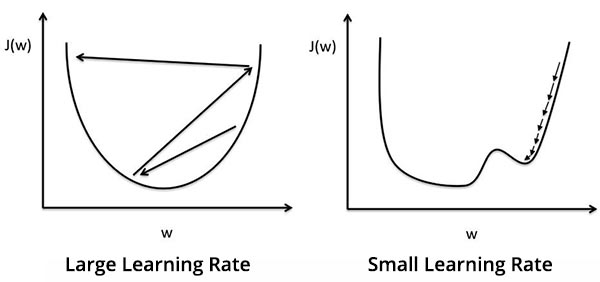In short, we use the gradient descent to find out the least cost for the function.

An algorithm for gradient descent: (repeat until convergence)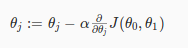where j=0,1 represents the feature index number, α is learning rate, J(theta0,theta1) is cost function.

If learning rate is too small, gradient descent can be slow. If learning rate is too large, gradient descent can overshoot the minimum. It may fail to converge and even diverge. At each iteration j, one should simultaneously update the parameters θ1,θ2,…,θn. Updating a specific parameter prior to calculating another one on the  iteration would yield to a wrong implementation.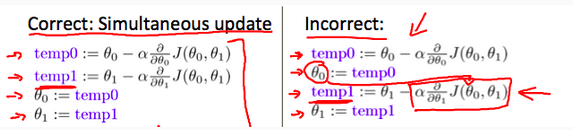In short, we use the gradient descent to find out the least cost for the function. Here, is the great answer from Parth Shah about the purpose for the use of gradient descent in machine learning.

Reference:

Andrew Ng, Machine learning Coursera

Some Useful Videos to Understand Gradient Descent:

## 3 thoughts on “What is Gradient Descent in machine learning?”

1.Pranav Sharma says:

2.Prabin Sharma says:
3.horloge says: